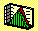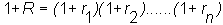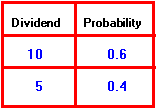2.2 PROBABILITY

There are (at least) two conceptual approaches to the concept of probability. For our purposes, they both lead to the same outcome.

The Empirical Approach

Suppose you flip a coin to decide between two courses of action. There are many possible outcomes that can occur. Upon landing, the coin may roll down a drain and become lost, or it may land on its edge. Only two of the many possible outcomes are relevant to the problem at hand: heads and tails. If the head turns up, then the first action is taken; if the tail turns up the second action is taken. Thus, if we put certain experimental controls in place to eliminate extraneous outcomes, we can conduct a coin flipping experiment, observe the outcomes, and see the random decisions made.

Sample Space

We can measure the observed outcome of the coin flipping experiment numerically, by representing a head as 1 and a tail as 0. This representation is convenient, because if we flip the coin twice, we can count the frequency of heads or tails occurring or we can represent the outcomes as points (e.g., (1,1), (1,0), (0,1), (0,0)) in two dimensions. Whatever the form, the set of points representing possible outcomes is called the sample space, or the event space, of the experiment.

Sample spaces provide a useful mathematical construct for building a theory of probability.

Assigning Probabilities to a Sample Space: Conceptual Considerations

Now consider repeating the coin toss experiment a great number of times. After n tosses, let x(n) be the frequency of heads, i.e., the number of heads observed divided by n. If the sample space is {0,1}, with 1 = heads and 0 = tails, then we can view x(n) as the probability of heads.

This construct allows us to give a probability, a physical interpretation, in terms of the relative frequency of a head or tail outcome occurring. This relative frequency number, which is between 0 and 1, is called a probability. The sum of these numbers over the set of possible outcomes equals one.

An applied statistician tends to view probabilities in this empirical or physical manner.

The Axiomatic Approach

Another conceptual approach to the concept of probability is the axiomatic treatment. It starts with a state space, S, and three axioms:

Axiom 1: To every state, s, is assigned a real number, p(s), greater than or equal to 0, called the probability of s.

Axiom 2: The sum of the probabilities equals 1.

Axiom 3: An event is a subset of the state space S. Given any event E, the probability of E is simply the "sum" of the probabilities of states in E.

The relative frequency interpretation of a probability satisfies these three axioms. In this case, what we call a sample space corresponds to a state space. Henceforth, we refer only to a state space.

Assigning Probabilities to a State Space: Operational Considerations

Operationally, there are two approaches to assigning probabilities to the states. These are linked to the two approaches taken to forecast future returns: --- the financial analyst approach and the financial statistician approach.

The first step for the financial analyst is to identify the state space. This requires classifying the economy into a discrete set of states. From the analyst's perspective, a state is a complete description of all the variables outside the market that affect the risk/return characteristics of an investment. For example, one state may include the demand for a firm's products, the cost of various inputs, and so on. The second step is to assign a probability to each state of the economy. This permits the return distribution to be constructed by combining this analysis with current market price information.

In the first step for the Three-Firm Case, all uncertainty arising from outside the market is summarized by ten states of the economy ranging from deep recession to strong expansion. In the second step each state is assessed to have an equal chance of occurring. You can review these steps in Chapter 1, topic 1.7, titled the Financial Analyst.

In contrast, the approach taken by the financial statistician is to infer the return distribution from a sample of observed return realizations. These returns are real numbers, and thus the state space is the set of these real numbers. The job of the financial statistician is to use historical returns to estimate the probability distribution of returns. This estimation is aided by results such as the central limit theorem, which allows this type of state characterization to be applied in statistical sampling. The financial statistician's approach is illustrated for the Three-Firm Case in Chapter 1, topic 1.8, titled the Financial Statistician.

Random Variables

More formally, a random variable is a function defined on a state space. The function that assigns probabilities to every possible event is a probability distribution. A probability distribution can either be discrete (if the random variable is a discrete outcome such as "heads" or "tails"), or continuous (if the random variable is a real numbered outcome such as an historic return (e.g., -0.02345)).

In the financial analyst approach, the end-of-period stock value is the random variable, which, given current prices, allows us to compute the return distribution.

In the financial statistician approach, the return is not only the state but is also the random variable (i.e., the random variable is the identity function).

Summary of State Space Probabilities

A frequently applied continuous probability distribution, is the normal or Gaussian distribution. This is a symmetric distribution that is defined over the real number line. It is different from the coin-toss example in that it is difficult to give it a physical interpretation. Instead it is completely specified by its mathematical form. This distribution is, however, of major theoretical and practical importance largely because of an important result from theoretical statistics called the central limit theorem. This theorem shows that the sample mean becomes normally distributed when your sample size goes to infinity (see central limit theorem).

This result suggests a theory of the relationship between intervals of normally distributed values and the probability of some naturally occurring random variable. You can apply the central limit theorem to the coin tossing experiment. You will see that even with only ten replications the normal distribution provides a useful practical assignment.

Coin Tossing Experiment: Normal Distribution Approximation

Suppose you record the outcome from ten tosses of a coin. Each toss results in either heads or tails. For N tosses the number or frequency of possible heads occurring is 0, 1, 2, ......, N - 1, N. The probability that the frequency of heads is 0, 1, 2, ......, N - 1, N can be computed exactly from the true underlying binomial probability distribution. These probabilities are provided in Table 2.1 for the case of ten tosses.

The normal approximations are also provided for N = 10. These are computed by first computing the standardized normal variable (Frequency - Mean)/(Standard Deviation). In this computation the mean and the standard deviation are from the underlying true binomial distribution (mean = 5, and standard deviation = 1.581). This standardized normal variable is referred to as the Z score. You can verify in Table 2.1 that the Z score associated with a frequency of heads equal to five (i.e., the frequency equals the mean) is zero. The probabilities are then computed for the interval around each Z score from the normal distribution, (see normal distribution), defined by the mid points between Z scores.

For example, the Z score for the frequency of heads equal to five is zero and the Z score for the frequency of heads equal to six (four) is 0.632 (-0.632). The midpoint between these two Z scores is 0.316 (-0.316) and therefore the interval is defined as [-0.316, 0.316].

Table 2.1

Coin Tossing Distribution

 Frequencyof Heads Z Score Binomial Probability Z Score Z Interval Cumulative Normal 0 0.00098 -3.162 +/- 0.316 0.002 1 0.00977 -2.530 +/- 0.316 0.011 2 0.04394 -1.897 +/- 0.316 0.044 3 0.01172 -1.265 +/- 0.316 0.115 4 0.20508 -0.632 +/- 0.316 0.204 5 0.24609 0.0 +/- 0.316 0.248 6 0.20508 0.632 +/- 0.316 0.204 7 0.01172 1.265 +/- 0.316 0.114 8 0.04394 1.897 +/- 0.316 0.044 9 0.00977 2.529 +/- 0.316 0.011 10 0.00098 3.162 +/- 0.316 0.002

You can see in Table 2.1 that a very good approximation is obtained for the exact binomial probabilities even with a sample size as small as 10.

Central Limit Theorem Applied to Returns

An interesting implication of the central limit theorem for investment theory is that, whatever the underlying true return distribution, with a large enough sample size (or, in other words, if the investment horizon is long enough), the normal distribution is a good approximation to the expected return (see financial statistician).

For example, suppose we assume that successive stock returns defined over some small period of time are independent and identically distributed over our investment horizon. Equivalently, we can view the realized 1 + return over the investment horizon as the product of n 1 + smaller period returns:The right-hand side of the 1 + R equation could be interpreted as daily returns over the investment horizon. By taking the natural logarithm of both sides, you get the realized return over the investment horizon equaling the sum of n daily returns. If the n daily returns are independent and identical realizations from some underlying return distribution (of any form with finite moments), and n is large, then the distribution of the ln(1 + R) is well approximated by the normal distribution. Furthermore, the natural log of the 1 + return over some period of time (ln(1 + R)) has a natural interpretation as the continuously compounded rate of return over the investment horizon.

Discrete Probability Distribution: An Investment Example

Assume a firm has 100 shares outstanding, and pays all its earnings to shareholders. Suppose there are two states, S = 2, and that the firm earns \$1,000 in state 1 and \$500 in state 2. Suppose further that the probability of state 1 is 0.6, and the probability of state 2 is 0.4. The earnings of the firm is a random variable. So is the dividend per share, which takes the value 10 in state 1, and the value 5 in state 2.

The discrete probability distribution for dividends is:More generally, we can let x(s) be the value of the random variable in state s and p(s) be the discrete probability distribution for x. In the investment example, x(s) could be either the earnings in state s or the dividend per share in state s. p(s) takes on the values of .6 and .4.

In modern portfolio theory important inputs to the optimal portfolio problem are statistics associated with the probability return distribution of financial securities --- in particular, the mean, variance, and covariance of returns of the financial securities. These statistical terms are well defined when the population return distribution is known (see topic 2.3, Population Statistics). Alternatively, population parameters can be estimated from a sample of past prices (see topic 2.4, Sample Statistics).

previous topic

next topic